# Log24

## Sunday, August 27, 2017

### Black Well

Filed under: General,Geometry — Tags: , — m759 @ 12:00 PM

The “Black” of the title refers to the previous post.
For the “Well,” see Hexagram 48.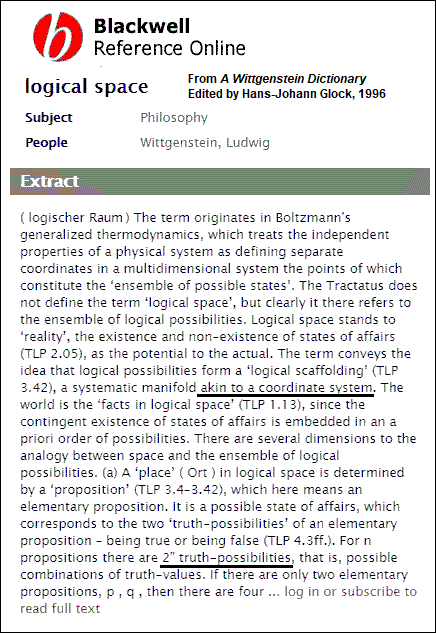Related material —

The Galois Tesseract and, more generally, Binary Coordinate Systems.

## Sunday, May 1, 2016

### Sunday Appetizer from 1984

Filed under: General,Geometry — Tags: — m759 @ 2:00 PM

Judith Shulevitz in The New York Times
on Sunday, July 18, 2010
(quoted here Aug. 15, 2010) —

“What would an organic Christian Sabbath look like today?”The 2015 German edition of Beautiful Mathematics ,
a 2011 Mathematical Association of America (MAA) book,
was retitled Mathematische Appetithäppchen —
Mathematical Appetizers . The German edition mentions
the author's source, omitted in the original American edition,
for his section 5.17, "A Group of Operations" (in German,
5.17, "Eine Gruppe von Operationen") —

 Mathematische Appetithäppchen: Faszinierende Bilder. Packende Formeln. Reizvolle Sätze Autor: Erickson, Martin — "Weitere Informationen zu diesem Themenkreis finden sich unter http://​www.​encyclopediaofma​th.​org/​index.​php/​ Cullinane_​diamond_​theorem und http://​finitegeometry.​org/​sc/​gen/​coord.​html ."

That source was a document that has been on the Web
since 2002. The document was submitted to the MAA
in 1984 but was rejected. The German edition omits the
document's title, and describes it as merely a source for
"further information on this subject area."

The title of the document, "Binary Coordinate Systems,"
is highly relevant to figure 11.16c on page 312 of a book
published four years after the document was written: the
1988 first edition of Sphere Packings, Lattices and Groups
by J. H. Conway and N. J. A. Sloane —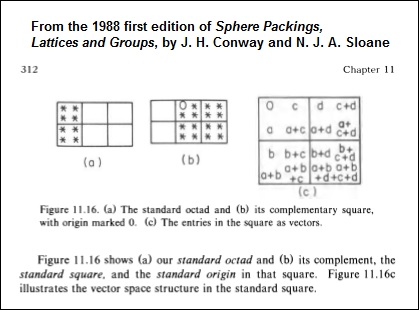A passage from the 1984 document —

## Thursday, June 6, 2013

### Review Comment Submitted

Filed under: General,Geometry — m759 @ 2:19 AM

The Mathematical Association of America has a
submit-a-review form that apparently allows readers
to comment on previously reviewed books.

This morning I submitted the following comment on
Alexander Bogomolny's March 16, 2012, review of
Martin J. Erickson's Beautiful Mathematics :

In section 5.17, pages 106-108, "A Group of Operations,"
Erickson does not acknowledge any source. This section
is based on the Cullinane diamond theorem. See that
theorem (published in an AMS abstract in 1979) at
PlanetMath.org and EncyclopediaOfMath.org, and
elsewhere on the Web. Details of the proof given by
Erickson may be found in "Binary Coordinate Systems,"
a 1984 article on the Web at
http://finitegeometry.org/sc/gen/coord.html.

If and when the comment may be published, I do not know.

Update of about 6:45 PM ET June 7:

The above comment is now online at the MAA review site.

Update of about 7 PM ET July 29:

The MAA review site's web address was changed, and the
above comment was omitted from the page at the new address.
This has now been corrected.

## Thursday, December 20, 2012

### Riddled

Filed under: General,Geometry — m759 @ 1:00 AM

See reference AR in "Binary Coordinate Systems."

See also Prewar Berlin in this journal.

## Wednesday, February 24, 2010

### Transvections

Filed under: General,Geometry — m759 @ 4:24 PM

A topic related to A Simple Reflection Group of Order 168

Transvection groups over GF(2). See, for instance…

1. Binary Coordinate Systems, by Steven H. Cullinane, 1984

2. Classification of the Finite N-Generator Transvection Groups Over Z2, by Jizhu Nan and Jing Zhao, 2009, Advances in Applied Mathematics Vol. 44 Issue 3 (March 2010), 185–202

3. Anne Shepler, video of a talk on Nov. 4, 2004, "Reflection Groups and Modular Invariant Theory"

## Friday, October 24, 2008

### Friday October 24, 2008

Filed under: General,Geometry — Tags: — m759 @ 8:08 AM

The Cube Space” is a name given to the eightfold cube in a vulgarized mathematics text, Discrete Mathematics: Elementary and Beyond, by Laszlo Lovasz et al., published by Springer in 2003. The identification in a natural way of the eight points of the linear 3-space over the 2-element field GF(2) with the eight vertices of a cube is an elementary and rather obvious construction, doubtless found in a number of discussions of discrete mathematics. But the less-obvious generation of the affine group AGL(3,2) of order 1344 by permutations of parallel edges in such a cube may (or may not) have originated with me. For descriptions of this process I wrote in 1984, see Diamonds and Whirls and Binary Coordinate Systems. For a vulgarized description of this process by Lovasz, without any acknowledgement of his sources, see an excerpt from his book.

## Wednesday, May 4, 2005

### Wednesday May 4, 2005

Filed under: General,Geometry — Tags: , — m759 @ 1:00 PM
The Fano Plane
Revisualized: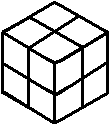or, The Eightfold Cube

Here is the usual model of the seven points and seven lines (including the circle) of the smallest finite projective plane (the Fano plane):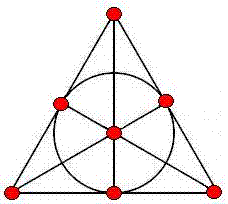Every permutation of the plane's points that preserves collinearity is a symmetry of the  plane.  The group of symmetries of the Fano plane is of order 168 and is isomorphic to the group  (See Cameron on linear groups (pdf).)

The above model indicates with great clarity six symmetries of the plane– those it shares with the equilateral triangle.  It does not, however, indicate where the other 162 symmetries come from.

Shown below is a new model of this same projective plane, using partitions of cubes to represent points: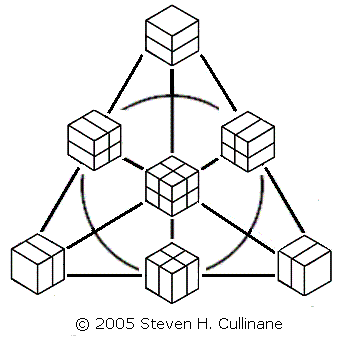The cubes' partitioning planes are added in binary (1+1=0) fashion.  Three partitioned cubes are collinear if and only if their partitioning planes' binary sum equals zero.

The second model is useful because it lets us generate naturally all 168 symmetries of the Fano plane by splitting a cube into a set of four parallel 1x1x2 slices in the three ways possible, then arbitrarily permuting the slices in each of the three sets of four. See examples below.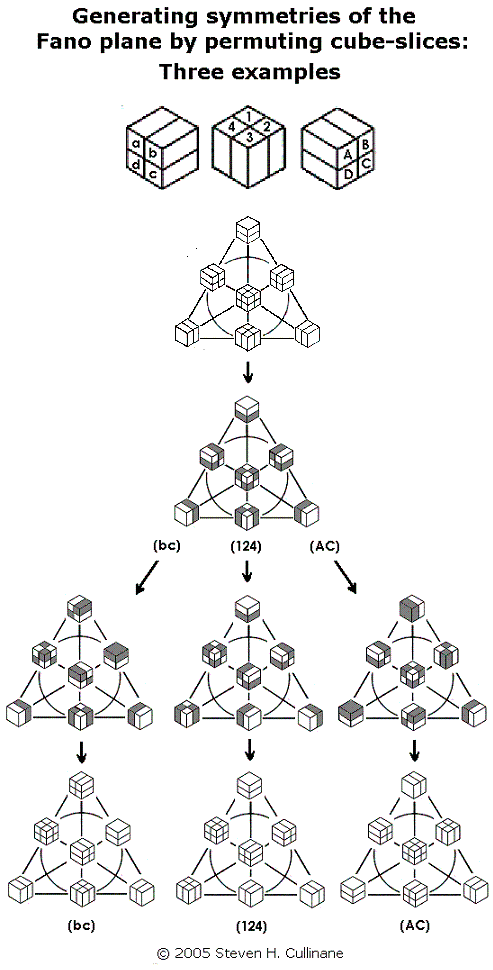For a proof that such permutations generate the 168 symmetries, see Binary Coordinate Systems.

(Note that this procedure, if regarded as acting on the set of eight individual subcubes of each cube in the diagram, actually generates a group of 168*8 = 1,344 permutations.  But the group's action on the diagram's seven partitions of the subcubes yields only 168 distinct results.  This illustrates the difference between affine and projective spaces over the binary field GF(2).  In a related 2x2x2 cubic model of the affine 3-space over GF(2) whose "points" are individual subcubes, the group of eight translations is generated by interchanges of parallel 2x2x1 cube-slices.  This is clearly a subgroup of the group generated by permuting 1x1x2 cube-slices.  Such translations in the affine 3-space have no effect on the projective plane, since they leave each of the plane model's seven partitions– the "points" of the plane– invariant.)

To view the cubes model in a wider context, see Galois Geometry, Block Designs, and Finite-Geometry Models.

For another application of the points-as-partitions technique, see Latin-Square Geometry: Orthogonal Latin Squares as Skew Lines.

For more on the plane's symmetry group in another guise, see John Baez on Klein's Quartic Curve and the online book The Eightfold Way.  For more on the mathematics of cubic models, see Solomon's Cube.

For a large downloadable folder with many other related web pages, see Notes on Finite Geometry.

## Saturday, July 20, 2002

### Saturday July 20, 2002

Filed under: General,Geometry — Tags: , — m759 @ 10:13 PM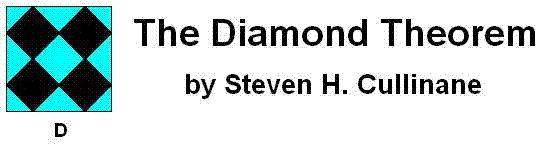ABSTRACT: Finite projective geometry explains the surprising symmetry properties of some simple graphic designs– found, for instance, in quilts. Links are provided for applications to sporadic simple groups (via the "Miracle Octad Generator" of R. T. Curtis), to the connection between orthogonal Latin squares and projective spreads, and to symmetry of Walsh functions.
We regard the four-diamond figure D above as a 4×4 array of two-color diagonally-divided square tiles.

Let G be the group of 322,560 permutations of these 16 tiles generated by arbitrarily mixing random permutations of rows and of columns with random permutations of the four 2×2 quadrants.

THEOREM: Every G-image of D (as at right, below) has some ordinary or color-interchange symmetry.

Example: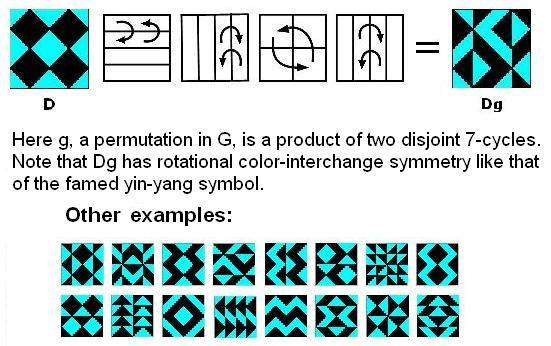For an animated version, click here. Remarks: Some of the patterns resulting from the action of G on D have been known for thousands of years. (See Jablan, Symmetry and Ornament, Ch. 2.6.) It is perhaps surprising that the patterns' interrelationships and symmetries can be explained fully only by using mathematics discovered just recently (relative to the patterns' age)– in particular, the theory of automorphism groups of finite geometries. Using this theory, we can summarize the patterns' properties by saying that G is isomorphic to the affine group A on the linear 4-space over GF(2) and that the 35 structures of the 840 = 35 x 24 G-images of D are isomorphic to the 35 lines in the 3-dimensional projective space over GF(2). This can be seen by viewing the 35 structures as three-sets of line diagrams, based on the three partitions of the four-set of square two-color tiles into two two-sets, and indicating the locations of these two-sets of tiles within the 4×4 patterns. The lines of the line diagrams may be added in a binary fashion (i.e., 1+1=0). Each three-set of line diagrams sums to zero– i.e., each diagram in a three-set is the binary sum of the other two diagrams in the set. Thus, the 35 three-sets of line diagrams correspond to the 35 three-point lines of the finite projective 3-space PG(3,2). For example, here are the line diagrams for the figures above: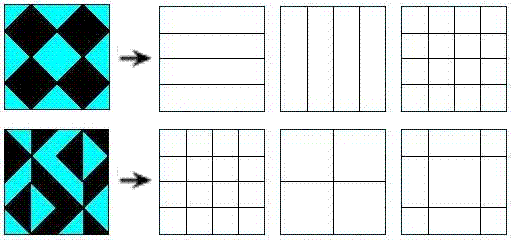Shown below are the 15 possible line diagrams resulting from row/column/quadrant permutations. These 15 diagrams may, as noted above, be regarded as the 15 points of the projective 3-space PG(3,2).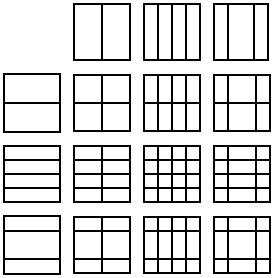The symmetry of the line diagrams accounts for the symmetry of the two-color patterns. (A proof shows that a 2nx2n two-color triangular half-squares pattern with such line diagrams must have a 2×2 center with a symmetry, and that this symmetry must be shared by the entire pattern.)

Among the 35 structures of the 840 4×4 arrays of tiles, orthogonality (in the sense of Latin-square orthogonality) corresponds to skewness of lines in the finite projective space PG(3,2). This was stated by the author in a 1978 note. (The note apparently had little effect. A quarter-century later, P. Govaerts, D. Jungnickel, L. Storme, and J. A. Thas wrote that skew (i.e., nonintersecting) lines in a projective space seem "at first sight not at all related" to orthogonal Latin squares.)

We can define sums and products so that the G-images of D generate an ideal (1024 patterns characterized by all horizontal or vertical "cuts" being uninterrupted) of a ring of 4096 symmetric patterns. There is an infinite family of such "diamond" rings, isomorphic to rings of matrices over GF(4).

The proof uses a decomposition technique for functions into a finite field that might be of more general use.

The underlying geometry of the 4×4 patterns is closely related to the Miracle Octad Generator of R. T. Curtis– used in the construction of the Steiner system S(5,8,24)– and hence is also related to the Leech lattice, which, as Walter Feit has remarked, "is a blown up version of S(5,8,24)."

For a movable JavaScript version of these 4×4 patterns, see The Diamond 16 Puzzle.

The above is an expanded version of Abstract 79T-A37, "Symmetry invariance in a diamond ring," by Steven H. Cullinane, Notices of the American Mathematical Society, February 1979, pages A-193, 194.

For a discussion of other cases of the theorem, click here.

Related pages:

 AMS Mathematics Subject Classification: 20B25 (Group theory and generalizations :: Permutation groups :: Finite automorphism groups of algebraic, geometric, or combinatorial structures) 05B25 (Combinatorics :: Designs and configurations :: Finite geometries) 51E20 (Geometry :: Finite geometry and special incidence structures :: Combinatorial structures in finite projective spaces)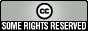This work is licensed under a
Creative Commons Attribution-NonCommercial-NoDerivs 2.5 License
.

Page created Jan. 6, 2006, by Steven H. Cullinane      diamondtheorem.com

Initial Xanga entry.  Updated Nov. 18, 2006.

Powered by WordPress